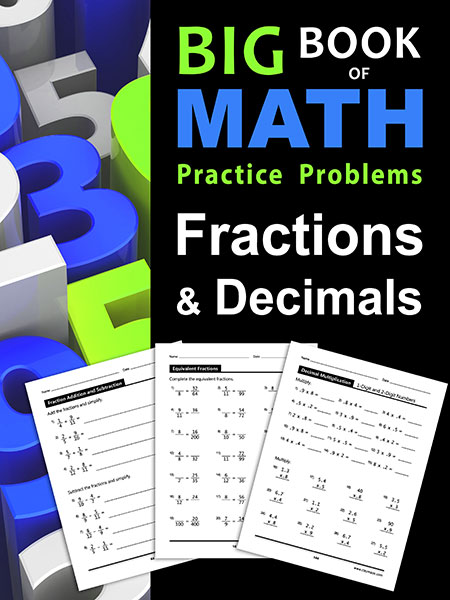### ClayMaze.com

Big Book of Math Practice Problems, Fractions and Decimals
Thousands of Fraction and Decimal Practice problems.  Solutions Included.

## Big Book of Math Practice Problems: Fractions and Decimals#### Big Book of Math Practice Problems Fractions and Decimals: Practice Workbook on Fractions and Decimals with Solutions